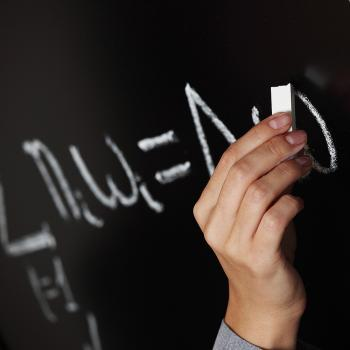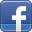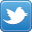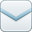In August of 2018, 65% of my students scored at or above the 50th percentile rank on the STAR Math Assessment. My goal is for 80% of my students to score at or above the 50th percentile rank on the STAR Math Assessment by May of 2018. This grant will help accomplish this goal by providing my class with three additional student laptop computers. These computers will be used during my math block to allow students extra remediation, practice, and enrichment by using math programs such as Zearn and Compass Learning. The primary benchmarks that will affect the math data are:

3.MD.2.4 Generate measurement data by measuring lengths using rulers marked with halves and fourths of an inch. Show the data
by making a line plot, where the horizontal scale is marked off in appropriate units-whole numbers, halves, or quarters.

3.NF.1.2.b Represent a fraction a/b on a number line diagram by marking off a lengths 1/b from 0. Recognize that the resulting
interval has size a/b and that its endpoint locates the number a/b on the number line.

3.MD.3.7.c Use tiling to show in a concrete case that the area of a rectangle with whole-number side lengths a and b + c is the sum
of a × b and a × c. Use area models to represent the distributive property in mathematical reasoning.

3.MD.3.7.b Multiply side lengths to find areas of rectangles with whole-number side lengths in the context of solving real world and
mathematical problems, and represent whole-number products as rectangular areas in mathematical reasoning.

3.MD.3.7.a Find the area of a rectangle with whole-number side lengths by tiling it, and show that the area is the same as would be
found by multiplying the side lengths.

3.MD.3.6 Measure areas by counting unit squares (square cm, square m, square in, square ft, and improvised units).

3.MD.3.5.b A plane figure which can be covered without gaps or overlaps by n unit squares is said to have an area of n square units.

3.MD.3.5.a A square with side length 1 unit, called "a unit square," is said to have "one square unit" of area, and can be used to
measure area.

< Back to Search Grants

# Empowering Math MindsSchool:
Van R Butler Elementary
Subject:
Low Performing Students
Teacher:
Sara Caudill
Students Impacted:
20
Grade:
Date:
September 29, 2018
Deadline:
May 31, 2019

### Investor

Thank you to the following investor for funding this grant.

The Alys Foundation - \$908.49

### Share

Please share this page to help in fulfilling this grant.### Impact to My Classroom

# of Students Impacted: 19

Thank you so much for supplying my classroom with 3 touchscreen laptops. My students were impacted daily! Our math scores increased as a result of this grant. Prior to the grant, 65% of my students were at or above the 50th percentile on STAR math. After the grant, 84% of my students were at or above the 50th percentile on STAR math!

## Original Grant Overview

### Goal

In August of 2018, 65% of my students scored at or above the 50th percentile rank on the STAR Math Assessment. My goal is for 80% of my students to score at or above the 50th percentile rank on the STAR Math Assessment by May of 2018. This grant will help accomplish this goal by providing my class with three additional student laptop computers. These computers will be used during my math block to allow students extra remediation, practice, and enrichment by using math programs such as Zearn and Compass Learning. The primary benchmarks that will affect the math data are:

3.MD.2.4 Generate measurement data by measuring lengths using rulers marked with halves and fourths of an inch. Show the data
by making a line plot, where the horizontal scale is marked off in appropriate units-whole numbers, halves, or quarters.

3.NF.1.2.b Represent a fraction a/b on a number line diagram by marking off a lengths 1/b from 0. Recognize that the resulting
interval has size a/b and that its endpoint locates the number a/b on the number line.

3.MD.3.7.c Use tiling to show in a concrete case that the area of a rectangle with whole-number side lengths a and b + c is the sum
of a × b and a × c. Use area models to represent the distributive property in mathematical reasoning.

3.MD.3.7.b Multiply side lengths to find areas of rectangles with whole-number side lengths in the context of solving real world and
mathematical problems, and represent whole-number products as rectangular areas in mathematical reasoning.

3.MD.3.7.a Find the area of a rectangle with whole-number side lengths by tiling it, and show that the area is the same as would be
found by multiplying the side lengths.

3.MD.3.6 Measure areas by counting unit squares (square cm, square m, square in, square ft, and improvised units).

3.MD.3.5.b A plane figure which can be covered without gaps or overlaps by n unit squares is said to have an area of n square units.

3.MD.3.5.a A square with side length 1 unit, called "a unit square," is said to have "one square unit" of area, and can be used to
measure area.

### What will be done with my students

By adding 3 laptops to our classroom, my 20 students will be able to spend at least 30 minutes per day on an individualized program to help them make learning gains in math. Students participate in math rotations which include teacher time, math fluency, technology, and math centers. During the technology rotation. Students will work for at least 30 minuted on one of the approved math programs.

### Benefits to my students

This year, 20 students will benefit from this grant. They will have more opportunities to work on individualized math programs. The focus of this grant will be the lowest 25% of my class. If my goal is reached, these students will benefit by growing in math from below to above the 50th percentile rank as evidenced by the STAR Math Assessment. Up to 20 students per year will benefit from this grant for as long as the laptops continue running efficiently.

### Budget Narrative

Items to be purchased:
4 Dell Latitude 3189 Touchscreen Laptops at \$299 each
2 6 Outlet Power Strips (Amazon) at \$11.49 for a 2-pack
Total = \$908.49

### Items

# Item Cost
1 Dell Latitude 3189 BTX 11.6in – Celeron N3350 – 4 GB RAM - 64 GB eMMC - 11.6" HD IPS 16:9 (1366 x 768) Touch with Corning® Gorilla® Glass NBT – Wireless-AC (TOUCHSCREEN) \$299.00
2 Dell Latitude 3189 BTX 11.6in – Celeron N3350 – 4 GB RAM - 64 GB eMMC - 11.6" HD IPS 16:9 (1366 x 768) Touch with Corning® Gorilla® Glass NBT – Wireless-AC (TOUCHSCREEN) \$299.00
3 Dell Latitude 3189 BTX 11.6in – Celeron N3350 – 4 GB RAM - 64 GB eMMC - 11.6" HD IPS 16:9 (1366 x 768) Touch with Corning® Gorilla® Glass NBT – Wireless-AC (TOUCHSCREEN) \$299.00
4 AmazonBasics 6-Outlet Surge Protector Power Strip 2-Pack, 200 Joule - Black \$11.49
Total: \$908.49

### Share

Please share this page to help in fulfilling this grant.